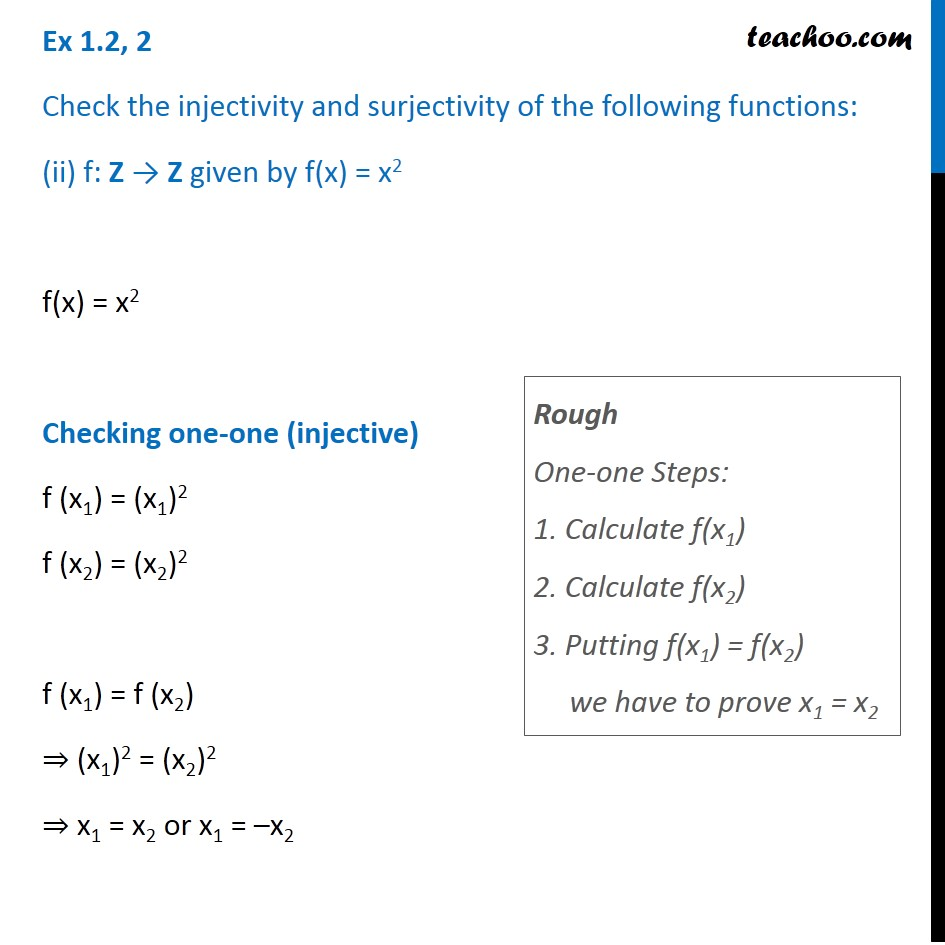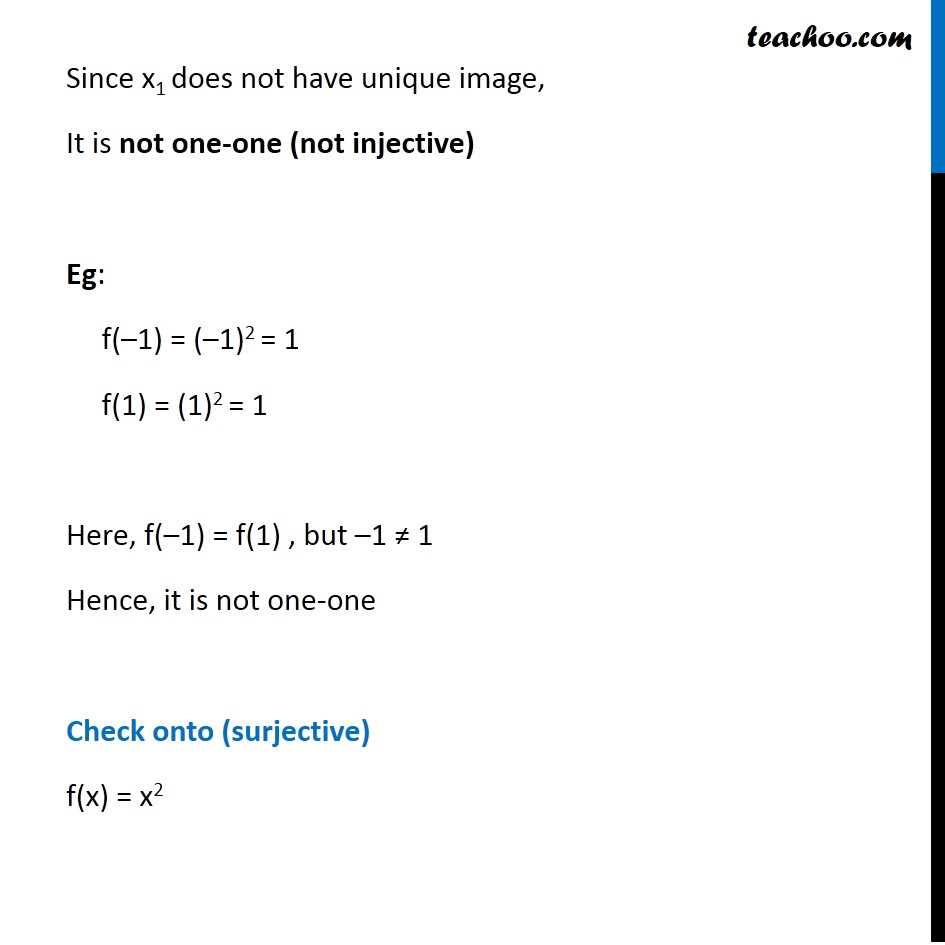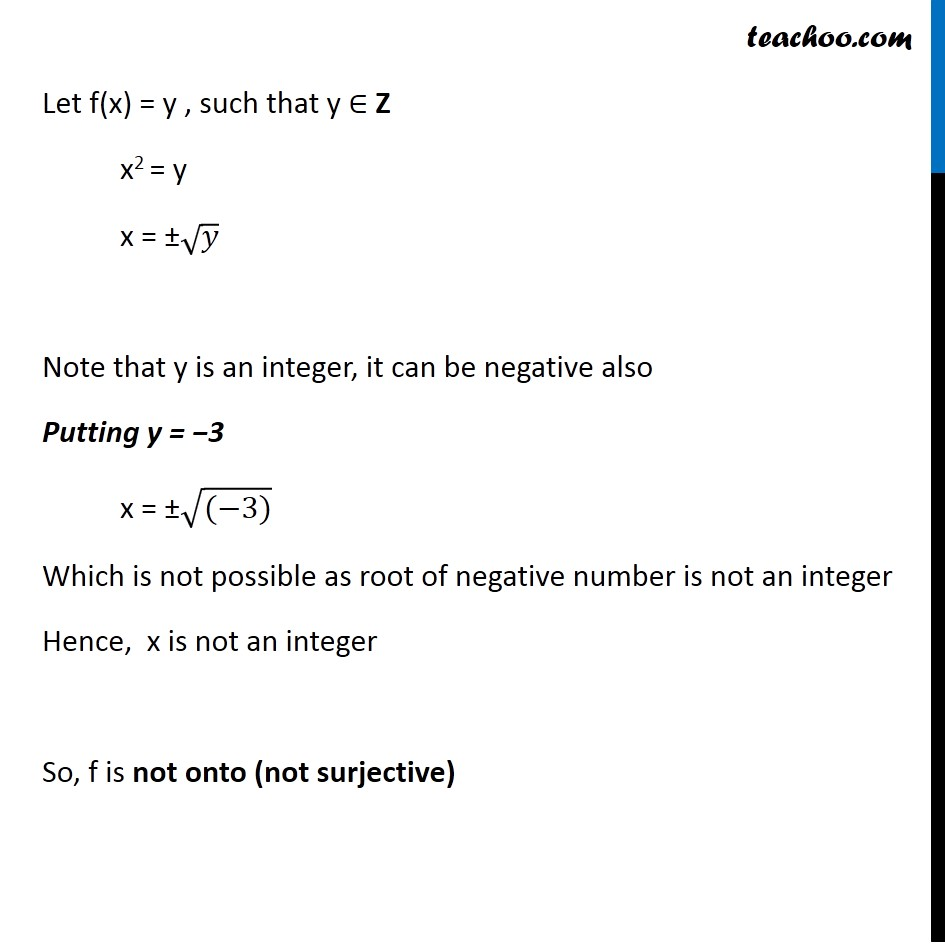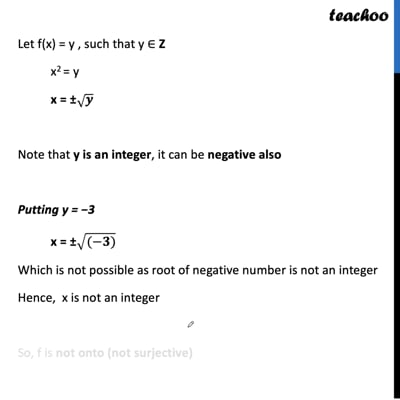Ex 1.2

Chapter 1 Class 12 Relation and Functions
Serial order wiseThis video is only available for Teachoo black users

### Transcript

Ex 1.2, 2 Check the injectivity and surjectivity of the following functions: (ii) f: Z → Z given by f(x) = x2 f(x) = x2 Checking one-one (injective) f (x1) = (x1)2 f (x2) = (x2)2 f (x1) = f (x2) ⇒ (x1)2 = (x2)2 ⇒ x1 = x2 or x1 = –x2 Rough One-one Steps: 1. Calculate f(x1) 2. Calculate f(x2) 3. Putting f(x1) = f(x2) we have to prove x1 = x2 Since x1 does not have unique image, It is not one-one (not injective) Eg: f(–1) = (–1)2 = 1 f(1) = (1)2 = 1 Here, f(–1) = f(1) , but –1 ≠ 1 Hence, it is not one-one Check onto (surjective) f(x) = x2 Let f(x) = y , such that y ∈ Z x2 = y x = ±√𝑦 Note that y is an integer, it can be negative also Putting y = −3 x = ±√((−3)) Which is not possible as root of negative number is not an integer Hence, x is not an integer So, f is not onto (not surjective)